# Hybridization of orbitals, 11 (1)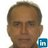Assistant Professor Chemical Engineering, Chemistry à Memorial University of Newfoundland
31 Mar 2020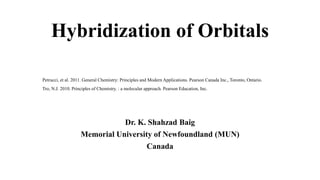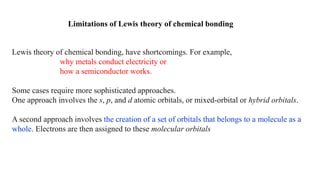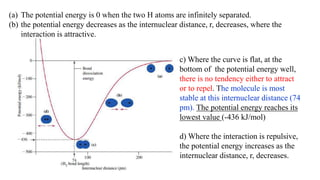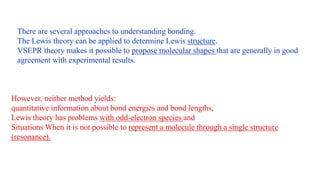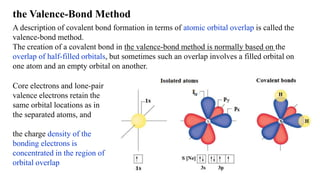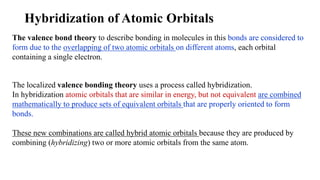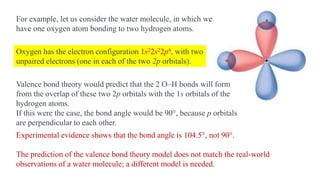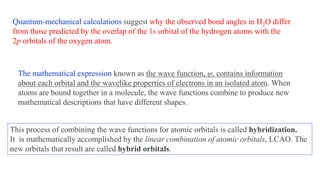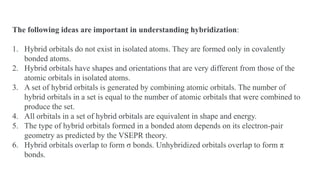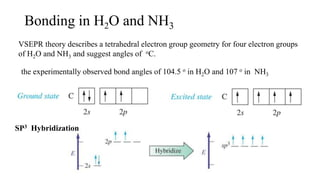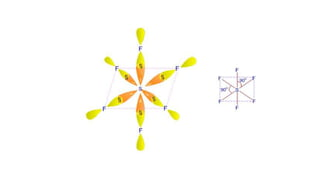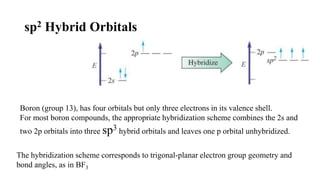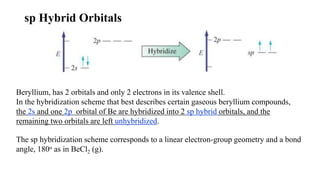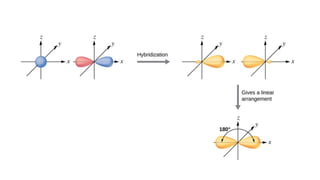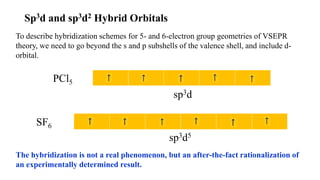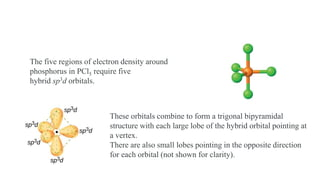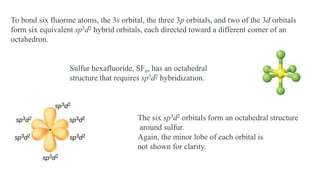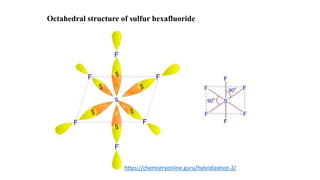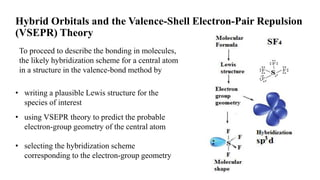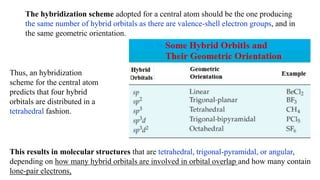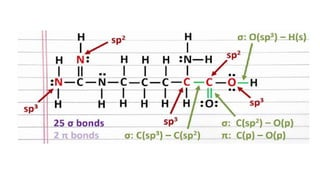1 sur 21

### Hybridization of orbitals, 11 (1)

• 1. Hybridization of Orbitals Dr. K. Shahzad Baig Memorial University of Newfoundland (MUN) Canada Petrucci, et al. 2011. General Chemistry: Principles and Modern Applications. Pearson Canada Inc., Toronto, Ontario. Tro, N.J. 2010. Principles of Chemistry. : a molecular approach. Pearson Education, Inc.
• 2. Lewis theory of chemical bonding, have shortcomings. For example, why metals conduct electricity or how a semiconductor works. Some cases require more sophisticated approaches. One approach involves the s, p, and d atomic orbitals, or mixed-orbital or hybrid orbitals. A second approach involves the creation of a set of orbitals that belongs to a molecule as a whole. Electrons are then assigned to these molecular orbitals Limitations of Lewis theory of chemical bonding
• 3. c) Where the curve is flat, at the bottom of the potential energy well, there is no tendency either to attract or to repel. The molecule is most stable at this internuclear distance (74 pm). The potential energy reaches its lowest value (-436 kJ/mol) d) Where the interaction is repulsive, the potential energy increases as the internuclear distance, r, decreases. (a) The potential energy is 0 when the two H atoms are infinitely separated. (b) the potential energy decreases as the internuclear distance, r, decreases, where the interaction is attractive.
• 4. There are several approaches to understanding bonding. The Lewis theory can be applied to determine Lewis structure. VSEPR theory makes it possible to propose molecular shapes that are generally in good agreement with experimental results. However, neither method yields: quantitative information about bond energies and bond lengths, Lewis theory has problems with odd-electron species and Situations When it is not possible to represent a molecule through a single structure (resonance).
• 5. A description of covalent bond formation in terms of atomic orbital overlap is called the valence-bond method. The creation of a covalent bond in the valence-bond method is normally based on the overlap of half-filled orbitals, but sometimes such an overlap involves a filled orbital on one atom and an empty orbital on another. Core electrons and lone-pair valence electrons retain the same orbital locations as in the separated atoms, and the charge density of the bonding electrons is concentrated in the region of orbital overlap the Valence-Bond Method
• 6. Hybridization of Atomic Orbitals The valence bond theory to describe bonding in molecules in this bonds are considered to form due to the overlapping of two atomic orbitals on different atoms, each orbital containing a single electron. The localized valence bonding theory uses a process called hybridization. In hybridization atomic orbitals that are similar in energy, but not equivalent are combined mathematically to produce sets of equivalent orbitals that are properly oriented to form bonds. These new combinations are called hybrid atomic orbitals because they are produced by combining (hybridizing) two or more atomic orbitals from the same atom.
• 7. Oxygen has the electron configuration 1s22s22p4, with two unpaired electrons (one in each of the two 2p orbitals). Valence bond theory would predict that the 2 O–H bonds will form from the overlap of these two 2p orbitals with the 1s orbitals of the hydrogen atoms. If this were the case, the bond angle would be 90°, because p orbitals are perpendicular to each other. For example, let us consider the water molecule, in which we have one oxygen atom bonding to two hydrogen atoms. Experimental evidence shows that the bond angle is 104.5°, not 90°. The prediction of the valence bond theory model does not match the real-world observations of a water molecule; a different model is needed.
• 8. Quantum-mechanical calculations suggest why the observed bond angles in H2O differ from those predicted by the overlap of the 1s orbital of the hydrogen atoms with the 2p orbitals of the oxygen atom. The mathematical expression known as the wave function, ψ, contains information about each orbital and the wavelike properties of electrons in an isolated atom. When atoms are bound together in a molecule, the wave functions combine to produce new mathematical descriptions that have different shapes. This process of combining the wave functions for atomic orbitals is called hybridization. It is mathematically accomplished by the linear combination of atomic orbitals, LCAO. The new orbitals that result are called hybrid orbitals.
• 9. The following ideas are important in understanding hybridization: 1. Hybrid orbitals do not exist in isolated atoms. They are formed only in covalently bonded atoms. 2. Hybrid orbitals have shapes and orientations that are very different from those of the atomic orbitals in isolated atoms. 3. A set of hybrid orbitals is generated by combining atomic orbitals. The number of hybrid orbitals in a set is equal to the number of atomic orbitals that were combined to produce the set. 4. All orbitals in a set of hybrid orbitals are equivalent in shape and energy. 5. The type of hybrid orbitals formed in a bonded atom depends on its electron-pair geometry as predicted by the VSEPR theory. 6. Hybrid orbitals overlap to form σ bonds. Unhybridized orbitals overlap to form π bonds.
• 10. Bonding in H2O and NH3 VSEPR theory describes a tetrahedral electron group geometry for four electron groups of H2O and NH3 and suggest angles of oC. the experimentally observed bond angles of 104.5 o in H2O and 107 o in NH3 SP3 Hybridization
• 12. sp2 Hybrid Orbitals Boron (group 13), has four orbitals but only three electrons in its valence shell. For most boron compounds, the appropriate hybridization scheme combines the 2s and two 2p orbitals into three sp3 hybrid orbitals and leaves one p orbital unhybridized. The hybridization scheme corresponds to trigonal-planar electron group geometry and bond angles, as in BF3
• 13. sp Hybrid Orbitals Beryllium, has 2 orbitals and only 2 electrons in its valence shell. In the hybridization scheme that best describes certain gaseous beryllium compounds, the 2s and one 2p orbital of Be are hybridized into 2 sp hybrid orbitals, and the remaining two orbitals are left unhybridized. The sp hybridization scheme corresponds to a linear electron-group geometry and a bond angle, 180o as in BeCl2 (g).
• 15. Sp3d and sp3d2 Hybrid Orbitals To describe hybridization schemes for 5- and 6-electron group geometries of VSEPR theory, we need to go beyond the s and p subshells of the valence shell, and include d- orbital. PCl5 sp3d SF6 sp3d5 The hybridization is not a real phenomenon, but an after-the-fact rationalization of an experimentally determined result.
• 16. The five regions of electron density around phosphorus in PCl5 require five hybrid sp3d orbitals. These orbitals combine to form a trigonal bipyramidal structure with each large lobe of the hybrid orbital pointing at a vertex. There are also small lobes pointing in the opposite direction for each orbital (not shown for clarity).
• 17. To bond six fluorine atoms, the 3s orbital, the three 3p orbitals, and two of the 3d orbitals form six equivalent sp3d2 hybrid orbitals, each directed toward a different corner of an octahedron. Sulfur hexafluoride, SF6, has an octahedral structure that requires sp3d2 hybridization. The six sp3d2 orbitals form an octahedral structure around sulfur. Again, the minor lobe of each orbital is not shown for clarity.
• 19. Hybrid Orbitals and the Valence-Shell Electron-Pair Repulsion (VSEPR) Theory To proceed to describe the bonding in molecules, the likely hybridization scheme for a central atom in a structure in the valence-bond method by • writing a plausible Lewis structure for the species of interest • selecting the hybridization scheme corresponding to the electron-group geometry • using VSEPR theory to predict the probable electron-group geometry of the central atom
• 20. The hybridization scheme adopted for a central atom should be the one producing the same number of hybrid orbitals as there are valence-shell electron groups, and in the same geometric orientation. Thus, an hybridization scheme for the central atom predicts that four hybrid orbitals are distributed in a tetrahedral fashion. This results in molecular structures that are tetrahedral, trigonal-pyramidal, or angular, depending on how many hybrid orbitals are involved in orbital overlap and how many contain lone-pair electrons,

### Notes de l'éditeur

1. We want simply to discover how these theories provide models that yield deeper insights into the nature of chemical bonding than do Lewis structures alone
2. Imagine bringing together two H atoms that are initially very far apart. When the H atoms are infinitely far apart, the two H atoms do not interact with each other, and by convention the net energy of interaction between the H atoms is zero. As the two H atoms approach each other, three types of interactions occur: each electron is attracted to the other nucleus the electrons repel each other (illustrated by a blue dashed (3) the two nuclei repel each At one particular internuclear distance (74 pm, condition c) the potential energy reaches its lowest value This is the condition in which the two H atoms combine into a molecule through a covalent bond. The nuclei continuously move back and forth; that is, the molecule vibrates, but the average internuclear distance remains constant. This internuclear distance corresponds to the bond length. The potential energy corresponds to the negative of the bond-dissociation energy. A theory of covalent bonding should help us understand why a given molecule has its particular set of observed properties bond-dissociation energies, bond lengths, bond angles, and so on
3. In looking at simple inorganic molecules such as H2 or HF, our present understanding of s and p atomic orbitals will suffice. In order to explain the bonding in organic molecules, however, we will need to introduce the concept of hybrid orbitals.
4. An sp3 hybrid orbital can also hold a lone pair of electrons. For example, the nitrogen atom in ammonia is surrounded by three bonding pairs and a lone pair of electrons directed to the four corners of a tetrahedron. The nitrogen atom is sp3 hybridized with one hybrid orbital occupied by the lone pair. The molecular structure of water is consistent with a tetrahedral arrangement of two lone pairs and two bonding pairs of electrons. Thus we say that the oxygen atom is sp3 hybridized, with two of the hybrid orbitals occupied by lone pairs and two by bonding pairs. Since lone pairs occupy more space than bonding pairs, structures that contain lone pairs have bond angles slightly distorted from the ideal. Perfect tetrahedra have angles of 109.5°, but the observed angles in ammonia (107.3°) and water (104.5°) are slightly smaller.  Unfortunately, however, recent experimental evidence indicates that in CH4 and NH3, the hybridized orbitals are not entirely equivalent in energy, making this bonding model an active area of research.
5. The issue of how best to describe the bonding orbitals in H2O and NH3 is still unsettled and underscores the occasional difficulty of finding a single theory that is consistent with all the available evidence. in the hybridization schemes of valence-bond theory, the number of orbitals is conserved; that is, in an sp2 hybridized atom there sp2 are still four orbitals: three hybrids and an unhybridized p orbital.
6. Boron s group 2 neighbor, beryllium,
7. Despite the difficulty posed by hybridization schemes involving d orbitals, the sp, sp2, and sp3 hybridization schemes are well established and very commonly encountered, particularly among the second-period elements